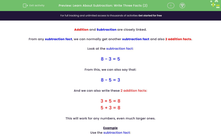# Learn About Subtraction: Write Three Facts (2)

In this worksheet, students look at a subtraction fact and write down three more facts based on it.Key stage:  KS 2

Curriculum topic:   Number: Addition and Subtraction

Curriculum subtopic:   Solve Add/Subtract Problems to 1000

Difficulty level:#### Worksheet Overview

From any subtraction fact, we can normally get another subtraction fact and also 2 addition facts.

Look at the subtraction fact:

8 - 3 = 5

From this, we can also say that:

8 - 5 = 3

And we can also write these 2 addition facts:

3 + 5 = 8

5 + 3 = 8

This will work for any numbers, even much larger ones.

Example

Use the subtraction fact:

63 - 27 = 36

to work out the values of A, B, C and D.

63 - 36 = A

36 + B = 63

27 + C = D

From the subtraction fact:

63 - 27 = 36

We get the other subtraction fact:

63 - 36 = 27

36 + 27 = 63

27 + 36 = 63

So

A = 27

B = 27

C = 36

D = 63

### What is EdPlace?

We're your National Curriculum aligned online education content provider helping each child succeed in English, maths and science from year 1 to GCSE. With an EdPlace account you’ll be able to track and measure progress, helping each child achieve their best. We build confidence and attainment by personalising each child’s learning at a level that suits them.

Get started